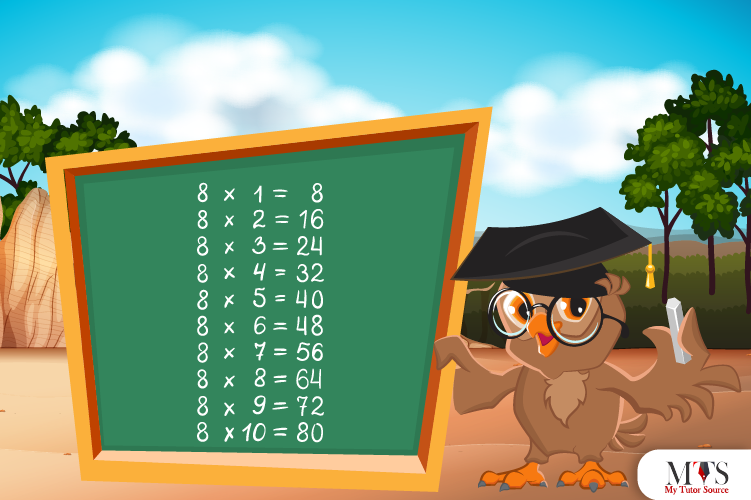# Multiplication Table of 8 – Tips to Memorize 8 Times Table & Example QuestionsAmong multiplication tables 1 – 20, students mostly struggle with 8 times table. They find its product confusing and intimidating as compared to other multiplication tables. Rote learning helps, but students learn better with exciting ways and worksheets. The tutoring approach of our online and private math tutors makes multiplication tables fun, easy to understand and memorize. Parents must look for innovative ways to help their kids grow academically in this rapidly changing world. In this article, we have given the 8 times table in mathematical form with the method to read and a few solved example questions using the 8 times table for quick learning.

## Table of 8

Following is the multiplication table of 8 up to 20. students must learn tables in chunks such as 8 times 5, then 8 times 10, and so on.

8 x 1 = 8
8 x 2 = 16
8 x 3 = 24
8 x 4 = 32
8 x 5 = 40
8 x 6 = 48
8 x 7 = 56
8 x 8 = 64
8 x 9 = 72
8 x 10 = 80
8 x 11 = 88
8 x 12 = 96
8 x 13 = 104
8 x 14 = 112
8 x 15 = 120
8 x 16 = 128
8 x 17 = 136
8 x 18 = 144
8 x 19 = 152
8 x 20 = 160

## Method to Read Table of 8

To learn, one must-read. Here, we have mentioned the method of reading 8 times table in words. Further, the method of reading a table in mathematics varies, but we have come up with the easiest way to read and learn. Check that:

Eight ones are eight (8)
Eight twos are sixteen (16)
Eight threes are twenty-four (24)
Eight fours are thirty-two (32)
Eight fives are forty (40)
Eight sixes are forty-eight (48)
Eight sevenths are fifty-six (56)
Eight eights are sixty-four (64)
Eight nines are seventy-two (72)
Eight tens are eighty (80)
Eight elevens are eighty-eight (88)
Eight twelves are ninety-six (96)
Eight thirteenths are one hundred and four (104)
Eight fourteens are one hundred and twelve (112)
Eight fifteens are one hundred and twenty (120)
Eight sixteenths are one hundred and twenty-eight (128)
Eight seventeens are one hundred and thirty-six (136)
Eight eighteens are one hundred and forty-four (144)
Eight nineteen are one hundred and fifty-two (152)
Eight twenty are one hundred and sixty (160)

## Tips for Memorizing Multiplication Table of 8

Memorizing the multiplication table of 8 boosts students’ confidence in their calculation skills, and they come up with strategies to tackle questions of home assignments and exams. Here are a few tips for memorizing the multiplication table of 8.

### Tip # 1: Spot the Pattern

Mathematics comes with the pattern, and so its multiplication tables. Kids who spot the pattern learn faster and master multiplication quickly. After the first half of the table, the pattern repeats itself in the other half. The multiples of 8 times table end with the digits 8, 6, 4, 2, and 0. Review the multiplication table of 8 and spot the pattern. It is a fun way to memorize this table quickly.

### Tip # 2: Repeated Addition

The product of 8 times table can be calculated with the repeated addition method. Students who are confident of their addition or sum skills must follow this method of memorizing the table. Below is the table for you:

### Tip # 3: Double the Table 4

The number 8 is the double of number 4, so its multiplication table is. It is one of the best tips for memorizing 8 times table as it helps students revise 4 times table. Let us see an example of doubling the table of 4.

For Example:

To find the answer of 8 times 8, we will find the answer of 4 times 8 and then double it. This is how,
4 x 8 = 32
After Doubling,
32 + 32 = 64
Hence, 8 x 8 = 64

### Example Question of 8 Times Table

Solution:

Given that,
Number of packets: 6
Number of cookies in each packet: 8
Therefore,
Total cookies = 6 x 8 = 48
Hence, Tommy will have 48 cookies in total.

#### Question: Write 8 times table from 5 to 10 (also in words)?

Following is the 8 times table from 5 to 10 both in mathematical form and words:

Question: Find the number just after 7 x 8?

Solution:

Using 8 times table,
7 x 8 = 56
Thus,
56 + 1 = 57
Hence, the number after 2 x 8 is 57

Question: Using a multiplication table of 8. Find out what 8 times 4 plus 8 times 9 is?

Solution:

Given that,
= 8 x 4 + 8 x 9
= 32 + 72
= 104
Hence, 8 times 4 plus 8 times 9 is equal to 104

Question: How many 8 are in 7 times 8? Answer with the product,

Solution:

7 x 8 = 8 + 8 + 8 + 8 + 8 + 8 + 8 + 8
Product = 7 x 8 = 56

### Find Top Tutors in Your AreaWith over 3 years of experience in teaching, Chloe is very deeply connected with the topics that talk about the educational and general aspects of a student's life. Her writing has been very helpful for students to gain a better understanding of their academics and personal well-being. I’m also open to any suggestions that you might have! Please reach out to me at chloedaniel402 [at] gmail.com